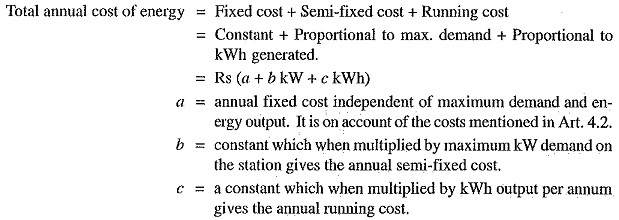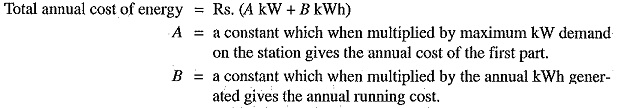## Cost of Electrical Energy:

The total Cost of Electrical Energy generated can be divided into three parts, namely ;

1. Fixed cost ;
2. Semi-fixed cost ;
3. Running or operating cost.

(i) Fixed cost: It is the cost which is independent of maximum demand and units generated.

The fixed cost is due to the annual cost of central organisation, interest on capital cost of land and salaries of high officials. The annual expenditure on the central organisation and salaries of high officials is fixed since it has to be met whether the plant has high Or low maximum demand or it generates less or more units. Further, the capital investment on the land is fixed and hence the amount of interest is also fixed.

(ii) Semi-fixed cost: It is the cost which depends upon maximum demand but is independent of units generated.

The semi-fixed cost is directly proportional to the maximum demand on power station and is on account of annual interest and depreciation on capital investment of building and equipment, taxes, salaries of management and clerical staff. The maximum demand on the power station determines its size and cost of installation. The greater the maximum demand on a power station, the greater is its size and cost of installation. Further, the taxes and clerical staff depend upon the size of the plant and hence upon maximum demand.

(iii) Running or operating cost: It is the cost which depends only upon the number of units generated.

The running cost is on account of annual cost of fuel, lubricating oil, maintenance, repairs and salaries of operating staff. Since these charges depend upon the energy output, the running cost is directly proportional to the number of units generated by the station. In other words, if the power station generates more units, it will have higher running cost and vice-versa.

### Expressions for Cost of Electrical Energy

The overall annual cost of electrical energy generated by a power station can be expressed in two forms viz three part form and two part form.

(i) Three part form. In this method, the overall annual cost of electrical energy generated is divided into three parts viz fixed cost, semi-fixed cost and running cost i.e.(ii) Two part form. It is sometimes convenient to give the annual cost of energy in two part form. In this case, the annual cost of energy is divided into two parts viz., a fixed sum per kW of maximum demand plus a running charge per unit of energy. The expression for the annual cost of energy then becomes :It is interesting to see here that two-part form is a simplification of three-part form. A little reflection shows that constant “a” of the three part form has been merged in fixed sum per kW maximum demand (i.e. constant A) in the two-part form.

Scroll to Top